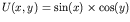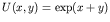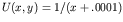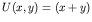Computational & Technology Resources an online resource for computational,engineering & technology publications not logged in - login Civil-Comp ProceedingsISSN 1759-3433 CCP: 84PROCEEDINGS OF THE FIFTH INTERNATIONAL CONFERENCE ON ENGINEERING COMPUTATIONAL TECHNOLOGY Edited by: B.H.V. Topping, G. Montero and R. Montenegro Paper 211A Numerical Comparison of Different Approximation Techniques in Meshfree Methods A. Emdadi1, N.A. Libre2, M. Rahimian2 and M. Shekarchi21Department of Civil Engineering, Islamic Azad University, Qazvin Branch, Tehran, Iran 2Department of Civil Engineering, University of Tehran, Tehran, Iran doi:10.4203/ccp.84.211 Full Bibliographic Reference for this paper A. Emdadi, N.A. Libre, M. Rahimian, M. Shekarchi, "A Numerical Comparison of Different Approximation Techniques in Meshfree Methods", in B.H.V. Topping, G. Montero, R. Montenegro, (Editors), "Proceedings of the Fifth International Conference on Engineering Computational Technology", Civil-Comp Press, Stirlingshire, UK, Paper 211, 2006. doi:10.4203/ccp.84.211 Keywords: approximation methods, meshfree, EFG, SPH, PIM, RBF. Summary There is a great interest in applying numerical methods for the solution of partial differential equations of various types of engineering problems. Numerical solution of partial differential equation consist of two main parts: approximating the solution of the equations (or unknown function) then determining the unknown function by embedding the approximated solution in the governing equation in the weak or strong form and applying boundary conditions to determine the unknown function. Numerical methods for the solution of partial differential equations have been well established over recent decades. In the last decade, researchers have paid attention to the meshless method without employing the concept of the element. Creation of a shape function is the central and most important issue in meshfree methods. The goal of this paper is comparing accuracy and efficiency of these different meshfree shape functions which have been proposed by researchers in the literature. In this paper five approximation methods is considered. The moving least squares (MLS) method which was originated by mathematicians for data fitting and surface construction. It can be categorized as a method of finite series representation of a function. Nayrolyes et al.  were the first to use MLS approximation to construct shape functions for their diffuse element method (DEM). The point interpolation approximation (PIM) using polynomial basis functions was originally developed by G.R. Liu et al.  and can be categorized as a finite series representation method. The SPH method that uses integral representation of a function and was presented by Lucy  for the first time. The radial basis function (RBF) approximation in which the approximation function depends only upon the radial distance between pairs of points. Its theoretical basis and convergence properties have been intensively studied during the last decade . The compactly supported RBF in which approximation function is locally defined . The accuracy of these methods for approximating simple mathematical functions including,,,and their derivatives were studied. Subsequently, the efficiency of different shape functions for approximating displacement and stress fields of elasticity problems consist of the patch test, the cantilever beam and a mode I crack opening was determined. The computational time used for approximation in each method was also studied. Numerical studies show that globally supported RBF performs excellently in approximating the displacement and stress field of different types of elasticity problem. But it is worth to note that this methods leads to a full and badly conditioned coefficient matrix. For engineering application, the CSRBF is recommended which reduces the computational effort required and numerical problems significantly. Using CSRBF makes the approximation faster than those based on globally supported RBF. On the other hand, this study shows that globally supported RBFs posses excellent accuracy, while the accuracy of the CSRBF is not so good. The accuracy of the CSRBF increases when increasing the support size. However, even when all data points are included in the support of an interpolation point, the accuracy of the CSRBF is still much worse than that of globally supported RBF. Therefore we suggest supplanting the CSRBF by the RBF and using other techniques such as domain decomposition to reduce the problems that are encountered in the numerical procedure. The approximate accuracy of the MLS method is very good but it is difficult to carry out and it takes so much time. The accuracy of the SPH method related to other methods in an elasticity problem is not good. In elasticity problems, the type of weight function in the MLS and SPH methods does not affect the accuracy seriously. References 1 B. Nayroles, G. Touzot, P. Villon, "Generalizing the Anite element method: diffuse approximation and diffuse elements", Journal of Computational Mechanics, 10, 307-318, 1992. doi:10.1007/BF00364252 2 G.R. Liu, Y.T. Gu, "A point interpolation method", Proceeding of 4th Asia-pacific Conference on Computational Mechanics, Singapore, 1009-014, 1999. 3 L.B. Lucy, "A numerical approach to the testing of the fission hypothesis", The Astronomical Journal, 82(12), 1013-1024, 1977. doi:10.1086/112164 4 M.J.D. Powell, "The theory of radial basis function approximation", Advances in numerical analysis, II: wavelets, subdivision algorithms and radial basis functions, Clarendon Press, 105-210, 1992. 5 Z. Wu, "Multivariate compactly supported positive definite radial basis functions", Journal of Advanced Computational Mathematics, 4, 283-292, 1995. doi:10.1016/0096-3003(94)00203-G purchase the full-text of this paper (price £20) Back to top ©Civil-Comp Limited 2020 - terms & conditions